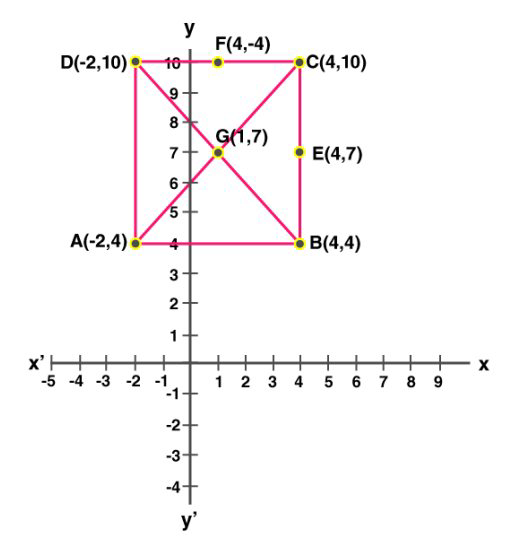Selina solutionsOur top 5% students will be awarded a special scholarship to Lido.
Set your child up for success with Lido, book a class today!

A (-2, 4), C(4, 10) and D(-2, 10) are the vertices of a square ABCD. Use the graphical method to find the coordinates of the fourth vertex B. Also, find:

(i) The coordinates of the mid-point of BC;

(ii) The coordinates of the mid-point of CD and

(iii) The coordinates of the point of intersection of the diagonals of the square ABCD.Looking to do well in your science exam ? Learn from an expert tutor. Book a free class!

Given , A (-2, 4), C(4, 10) and D(-2, 10) are the vertices of a square ABCDAccording to the graph, we get, B(4,4)

From the graph,

The coordinates of the mid-point of BC is E(4,7)

The coordinates of the mid-point of CD is F(1,10)

the coordinates of the diagonals of the square is G(1,7)

Our top 5% students will be awarded a special scholarship to Lido.Lido

Courses

Teachers

Book a Demo with us

Syllabus

Science
English
Coding

Terms & Policies

NCERT Question Bank

Maths
Science

Selina Question Bank

Maths
Physics
Biology

Allied Question Bank

Chemistry